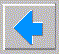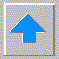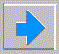# Lecture 2 - Pattern Recognition

• The simplest type of AI - Pattern Recognition
• classification of real-world objects
• not a unified field, more art than science
• Not a trivial problem
• example: complete the sequence 1, 2, 3, 4, 5, 6, 7, X
• desired answer: 5048 since the sequence was n + (n-1)(n-2)(n-3)(n-4)(n-5)(n-6)(n-7)
• Occam's razor is hard to explain to a computer
• A few applications:
• speech and speaker recognition
• character or signature recognition
• image and scene recognition
• fingerprint recognition
• biomedical applications
• recognition can be from closed set (verification) or open set (spotting)
• for verification there is probability of correct classification
• for spotting there is also false alarm rate (trade-off)
• Syntactical (Linguistic) Approach (GOFAI)
• Describe classes by rules (grammar) in a formal language
• Identify pattern's class by the grammar it obeys
• like identifying computer language by trying different compilers
• Reduces classification to string parsing
• Typical applications: Fingerprinting, Scene analysis, Chinese character recognition
• Statistical (Decision Theoretic) Approach
• Describe patterns as points in n dimensional vector space
• Describe classes as hypervolumes or statistical clouds
• Reduces classification to geometry or function evaluation
• Typical applications: Signals, Speech, Latin character recognition
• Comparison:
•                    Class A            Class B            Class C
Statistical  (1,   0,   0  )    (0,   1,    0  )   (0,   0,   1  )
(0.9, 0.1, 0  )    (0.1, 1,   -0.1)  (-0.1, 0.1, 1  )
(1.1, 0,   0.1)    (0,   0.9, -0.1)  ( 0.1, 0,   1.1)

Syntactic      (1, 1, 1)          (1, 2, 3)          (1, 2, 4 )
(2, 2, 2)          (2, 3, 4)          (2, 4, 8 )
(3, 3, 3)          (3, 4, 5)          (3, 6, 12)

• How hard can it be?
• small spheres far apart
• large spheres close together
• rotated cigars
• bananas
• true overlap
• Bayes Decision Theory
• priors - a priori probability distribution p(x|ci)
• posteriors - classify by MAP
• Bayes theorem: p(x, ci) = p(ci|x) p(x) = p(x|ci) p(ci)
• so p(ci|x) = p(ci) p(x|ci) / p(x) where p(x) = \sum_i p(ci) p(x|ci)
• Bayes error is the minimal error (draw 2 Gaussians w/ overlap)
• Why do we need anything else ? unknown priors!
• So does pattern recognition reduce to estimating priors?
• No - generalization (remember real-world)
• Learning Theory
• Supervised
• Unsupervised (clustering)
• Reinforcement (good dog, bad dog)
• Cross Validation
• Training set vs. test set
• Validation set
• Training error
• Test error
• Structure of classical pattern recognition system
• Preprocessing (noise reduction, registration, etc.)
• Feature extraction (dimensionality reduction)
• Classification
• Postprocessing (smoothing, outlier removal, etc.)
• Generic classifiers (from more direct probability estimate to more separation)
• Direct probability estimation (1NN, kNN, Parzen; LBG, LVQ)
• Hypersphere (potentials, Mahalanobis, RBF, RCE)
• Hyperplane (KL, Fisher, CART, MLP)
• The VC revolution
• PAC (Probably Approximately Correct) Learning
• teacher and student - same prob dist from world
• limit on generalization error p(E>e) < 1 - d
• VC dimension - strength of classifier
• 1d with line d=2
• 2d line d=3
• 2d arbitrary rect d=4
• Match classifier's VC dimension to amount of training data
• Egen - Etrain < dVC / Ntrain